# rxjs入门6之合并数据流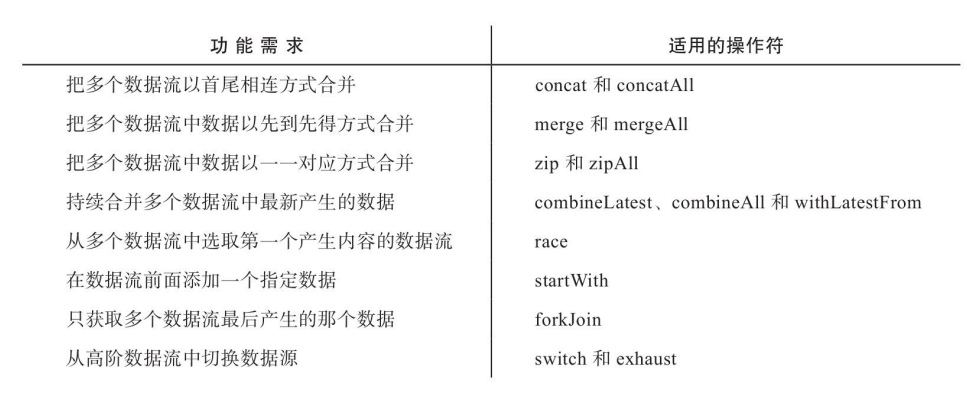### 一 concat，merge，zip，combineLatest等合并类操作符

import {concat,merge,zip,combineLatest}
##### 1.concat (obs1,obs2,obs3) 首尾相连

concat(of(1,2,3),interval).subscribe(console.log);
// 1   2   3    0  1  2  3  ...
##### 2.merge 先到先得快速通过

merge会第⼀时间订阅所有的上游Observable，然后对上游的数据采取“先到先得”的策略，任何⼀个Observable只要有数据推下来，就⽴刻转给下游Observable对象。

merge(interval(1000),of(1,2,3)).subscribe(console.log);
merge(of(1,2,3),interval(1000)).subscribe(console.log);
//两种情况的输出结果一样，都是先一次性输出1 2 3  再间隔一秒依次输出0 1 2 ...
const source1$= Observable.timer(0, 1000).map(x => x+'A'); const source2$ = Observable.timer(500, 1000).map(x => x+'B');
merge(source1$, source2$).subscribe(
console.log,
null,
() => console.log('complete')
);
//0A
//0B
//1A
//1B
//2A
//2B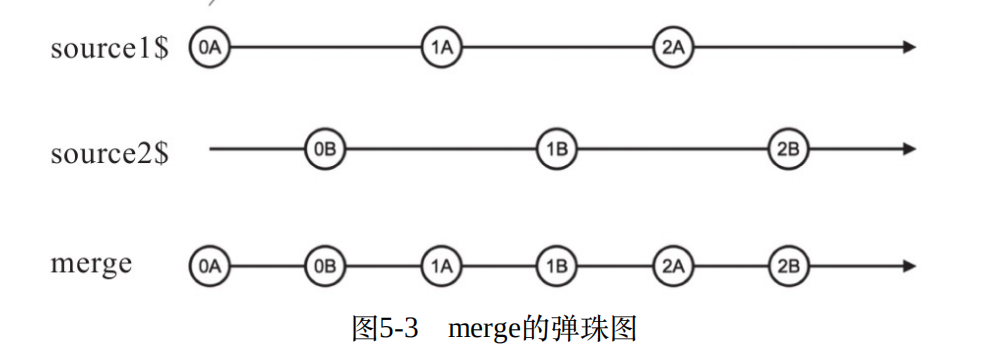merge 的应用场景：我们知道fromEvent可以从⽹页中获取事件，只可惜，fromEvent⼀次只能从⼀个DOM元素获取⼀种类型的事件。⽐如，我们关⼼某个元素的click事件，同时也关⼼这个元素上的touchend事件，因为在移动设备上touchend事件出现得⽐click更早，这两个事件的处理是⼀模⼀样的，但是fromEvent不能同时获得两个事件的数据流，这时候就要借助merge的⼒量了，代码如下：

const click$= Rx.Observable.fromEvent(element, 'click'); const touchend$ = Rx.Observable.fromEvent(element, 'touchend');
merge(click$, touchend$).subscribe(eventHandler)
##### 3.zip :拉链式组合

• zip会把上游的数据转化为数组形式，每⼀个上游Observable贡献的数据会在对应数组中占⼀席之地.
• 默认的输出格式为数组格式，可通过第二个参数进行参数格式组装
• 简而言之：不管是同步产生数据还是异步产生的数据，都会每次依次从需要合并的observable中取一个数据合并成一个数组输出，当某一个observer不再吐出数据了，则终止合并，执行complete函数
const source1$= Observable.of(1, 2, 3); const source2$ = Observable.of('a', 'b', 'c');
zip(source1$, source2$).subscribe(
console.log,
null,
() => console.log('complete')
);
//[ 1, 'a' ]
//[ 2, 'b' ]
//[ 3, 'c' ]
//complete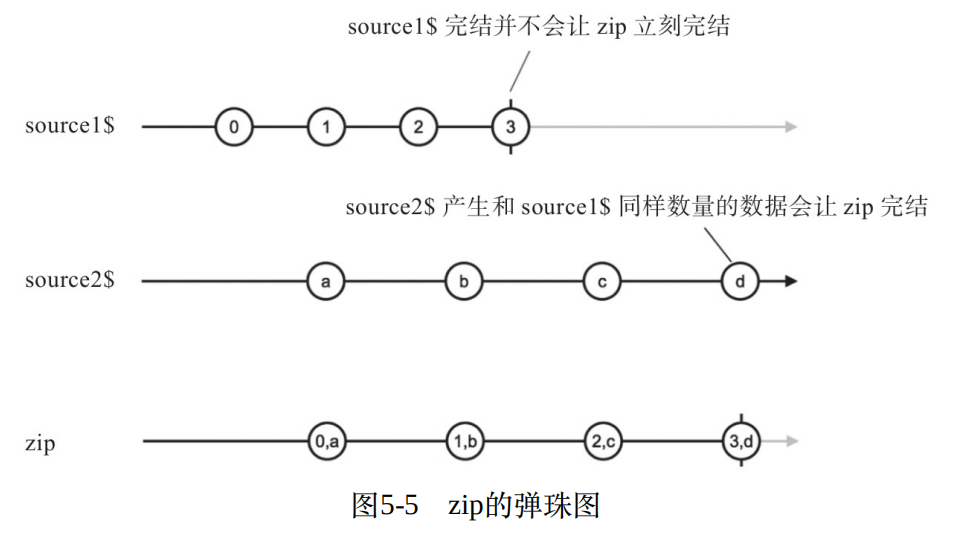##### 4.combineLatest:合并最后一个数据
• 输出的数组中元素个数与合并的observable的个数相等。
• 在合并的observable中，只有最后一个元素为下游，前面的参数如果有同步的数据，同步数据中只有最后一个数据能进入数据流
• 默认的输出格式为数组格式，可通过第二个参数进行参数格式组装
• 第一次执行，当上游产生了数据，下游还没来得及产生数据时，就会等待。第二轮时候，不管是上游或者下游产生一个数据，都会执行输出，还没来得及产生数据的observable就输出原来产生的数据，如下弹珠图
const source1$= Observable.timer(500, 1000); const source2$ = Observable.timer(1000, 1000);
combineLatest(source1$,source2$).subscribe(
console.log,
null,
() => console.log('complete')
);
//[ 0, 0 ]
//[ 1, 0 ]
//[ 1, 1 ]
//[ 2, 1 ]
//[ 2, 2 ]
//[ 3, 2 ]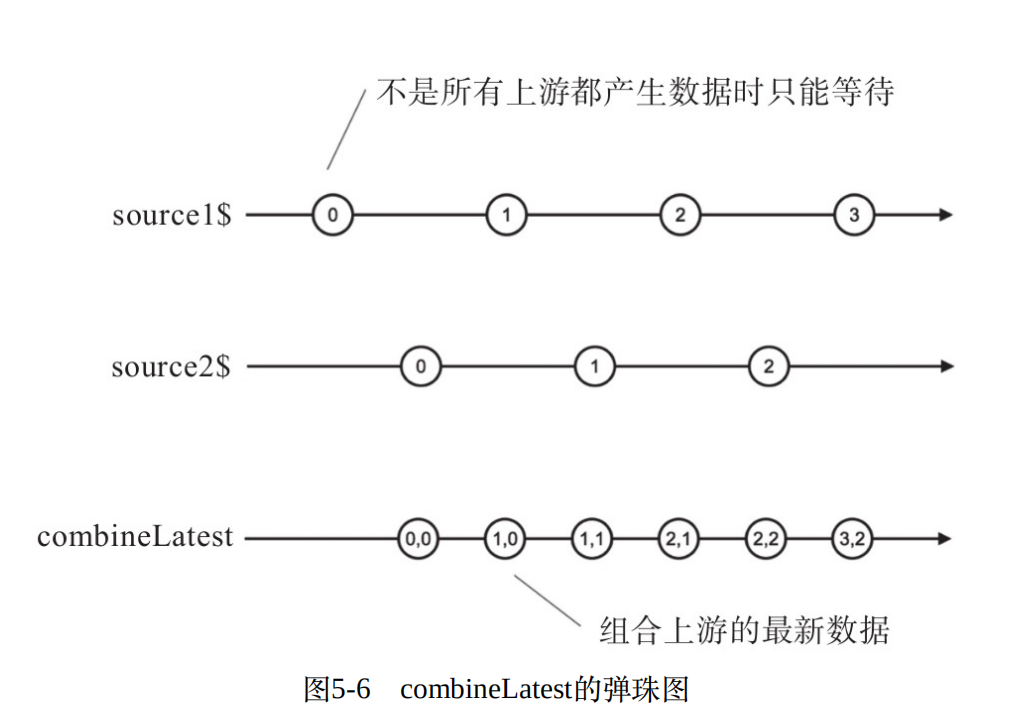##### 5.withLatestFrom
• withLatestFrom 为管道中使用的方法。默认的输出格式为数组格式，可通过第二个参数进行参数格式组装
• withLatestFrom只有实例操作符的形式，⽽且所有输⼊Observable的地位并不相同，调⽤withLatestFrom的那个Observable对象起到主导数据产⽣节奏的作⽤，作为参数的Observable对象只能贡献数据，不能控制产⽣数据的时机。
const source1$= Observable.timer(0, 2000).map(x => 100 * x); const source2$ = Observable.timer(500, 1000);
source1$.pipe( withLatestFrom(source2$, (a,b)=> a+b);
).subscribe(
console.log,
null,
() => console.log('complete')
);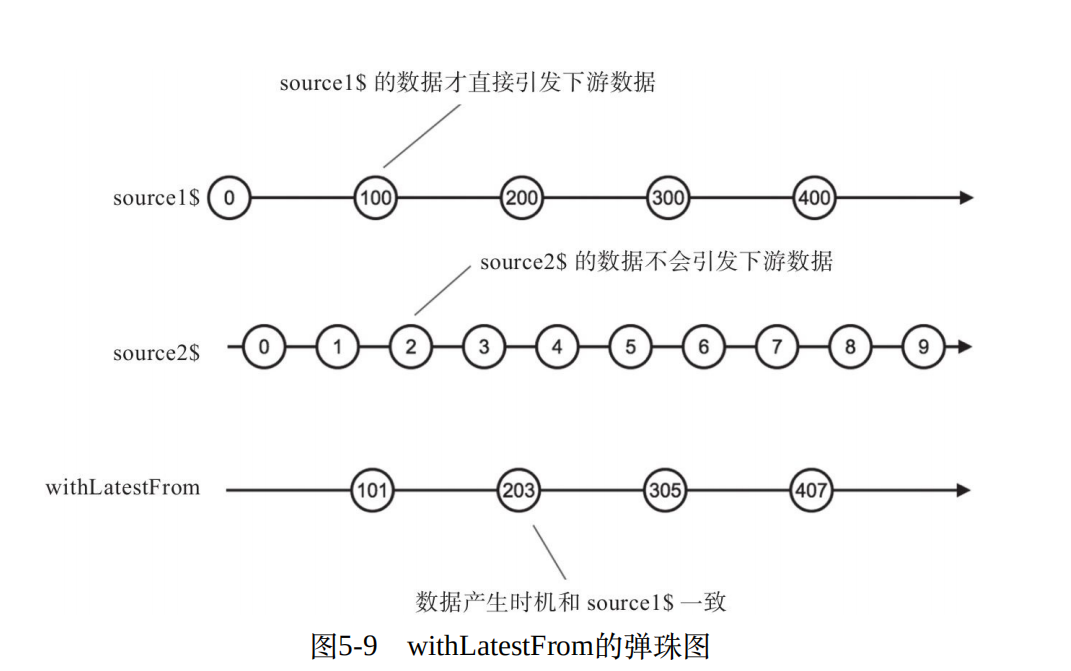source1$产⽣第⼀个数据0时，withLatestFrom的另⼀个输⼊Observable对象source2$还没有产⽣数据，所以这个0也被忽略了。

const original$= Observable.timer(0, 1000); const source1$ = original$.map(x => x+'a'); const source2$ = original$.map(x => x+'b'); const result$ = source1$.pipe(withLatestFrom(source2$);)
result$.subscribe( console.log, null, () => console.log('complete') ); 例2： const event$ = Rx.Observable.fromEvent(document.body, 'click');
const x$= event$.map(e => e.x);
const y$= event$.map(e => e.y);
const result$= x$.pipe(combineLatest(y$, (x, y) => x:${x}, y: ${y})).subscribe( (location) => { console.log('#render', location); document.querySelector('#text').innerText = location; } ); ##### race :胜者通吃 race就是“竞争”，多个Observable对象在⼀起，看谁最先产⽣数据，不过这种竞争是⼗分残酷的，胜者通吃，败者则失去所有机会。 简而言之，通过race合并多个observable时，最先吐出数据那个observable会成为数据源，其它的observable会被淘汰。 ##### startWith startWith只有实例操作符的形式，其功能是让⼀个Observable对象在被订阅的时候，总是先吐出指定的若⼲个数据。下⾯是使⽤startWith的⽰例代码 of(0,1,2).pipe(startWith('a','b')).subscribe(console.log); //先依次吐出 a b 0 1 2 ##### forkJoin • forkJoin可以接受多个Observable对象作为参数，forkJoin产⽣的Observable对象也很有特点，它只会产⽣⼀个数据，因为它会等待所有参数Observable对象的最后⼀个数据，也就是说，只有当所有Observable对象都完结，确定不会有新的数据产⽣的时候，forkJoin就会把所有输⼊Observable对象产⽣的最后⼀个数据合并成给下游唯⼀的数据。 • forkJoin就是RxJS界的Promise.all，Promise.all等待所有输⼊的Promise对象成功之后把结果合并，forkJoin等待所有输⼊的Observable对象完结之后把最后⼀个数据合并。 • 返回数组形式，数组中元素个数为合并的observable的个数 js forkJoin(interval(1000).pipe(take(3)),of(1,2,3),timer(2000,1000).pipe(take(3))).subscribe(console.log); // [2,3,2]js ### 高阶Observable 简言之：⾼阶函数就是产⽣函数的函数；类似，所谓⾼阶Observable，指的是产⽣的数据依然是Observable的Observable ##### 1.concatAll concatAll只有⼀个上游Observable对象，这个Observable对象预期是⼀个⾼阶Observable对象，concatAll会对其中的内部Observable对象做concat的操作. interval(1000).pipe( take(2), map(x=>interval(1500).pipe(take(2),map(x=> ${x}:x,y:\${y}))),
concatAll()
).subscribe(console.log);
// 0:a,b:0
// 0:a,b:1
// 1:a,b:0
// 1:a,b:1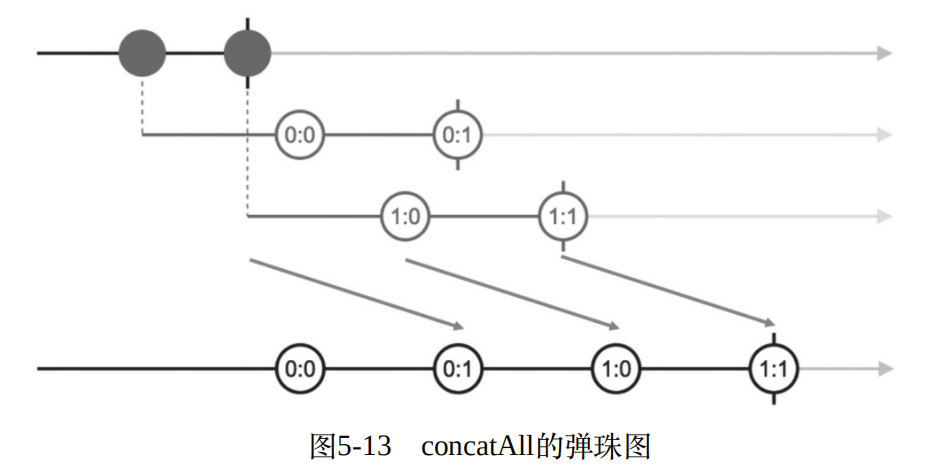concat 实际运用

fromEvent(document.body,'mousedown').pipe(
map(
e=>fromEvent(document.body,'mousemove').pipe(map(e=>{return {x:e.clientX,y:e.clientY}}), takeUntil(fromEvent(document.body,'mouseup')))
),
concatAll()
).subscribe(console.log);
##### mergeAll

mergeAll就是处理⾼阶Observable的merge，只是所有的输⼊Observable来⾃于上游产⽣的内部Observable对象.

interval(1000).pipe(
take(2),
map(x => Observable.interval(1500).map(y => x+':'+y).take(2)),
mergeAll()
)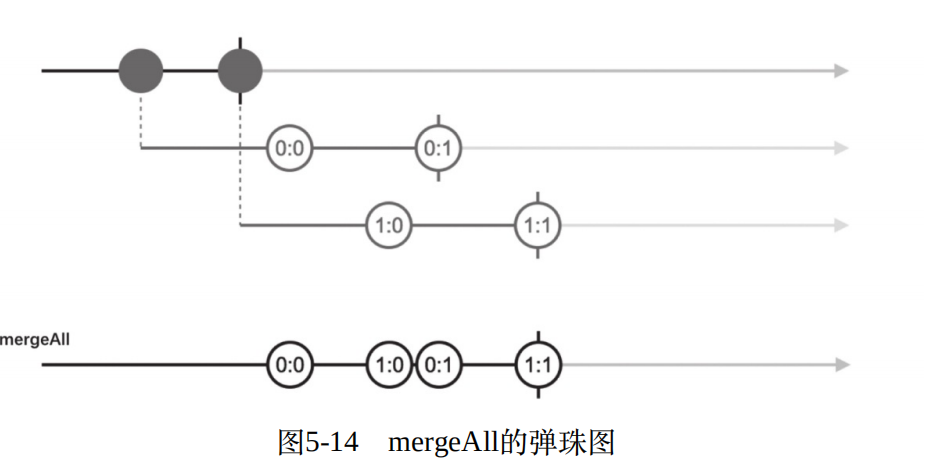mergeAll只要

##### zipAll
interval(1000).pipe(
take(2),
map(x => Observable.interval(1500).map(y => x+':'+y).take(2)),
zipAll()
)
//[ '0:0', '1:0' ]
//[ '0:1', '1:1' ]
//complete
##### combineAll

combineAll就是处理⾼阶Observable的combineLatest，可能是因为combine-LatestAll太长了，所以RxJS选择了combineAll这个名字。

interval(1000).pipe(
take(2),
map(x => Observable.interval(1500).map(y => x+':'+y).take(2)),
combeneAll()
)
//[ '0:0', '1:0' ]
//[ '0:1', '1:0' ]
//[ '0:1', '1:1' ]
//complete
##### switchAll
• switch的含义就是“切换”，总是切换到最新的内部Observable对象获取数据。每当switch的上游⾼阶Observable产⽣⼀个内部Observable对象，switch都会⽴刻订阅最新的内部Observable对象上，如果已经订阅了之前的内部Observable对象，就会退订那个过时的内部Observable对象，这个“⽤上新的，舍弃旧的”动作，就是切换。
• 应用场景：也就是外层的数据产生快于内层的数据产生的速度造成数据积压，需求又能够舍弃原来的旧的外层的数据不让其旧的外层数据再传递到内层产生数据了。
简而言之，当外层新产生数据时，无论内部数据产生情况如何都作废，重新计算数据流
interval(1000).pipe(
take(3),
map(x => Observable.interval(1500).map(y => x+':'+y).take(2)),
switchAll()
)
//1:0
//1:1
//complete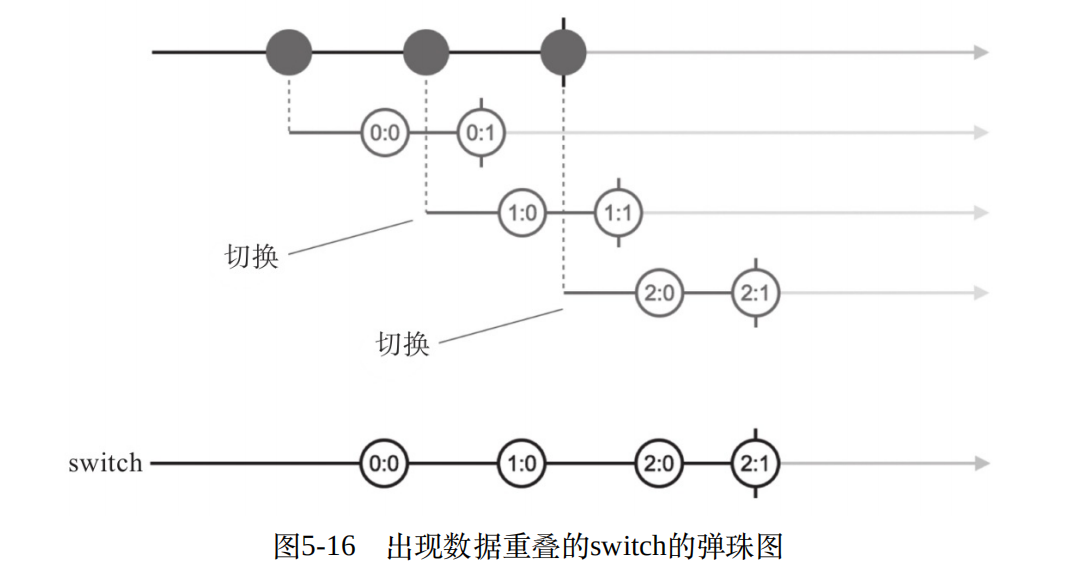##### exhaust
• 在耗尽当前内部Observable的数据之前不会切换到下⼀个内部Observable对象
• 同样是连接⾼阶Observable产⽣的内部Observable对象，但是exhaust的策略和switch相反，当内部Observable对象在时间上发⽣重叠时，情景就是前⼀个内部Observable还没有完结，⽽新的Observable又已经产⽣，到底应该选择哪⼀个作为数据源？switch选择新产⽣的内部Observable对象，exhaust则选择前⼀个内部Observable对象.
interval(1000).pipe(
take(3),
map(x => Observable.interval(700).map(y => x+':'+y).take(2)),
exhaust()
)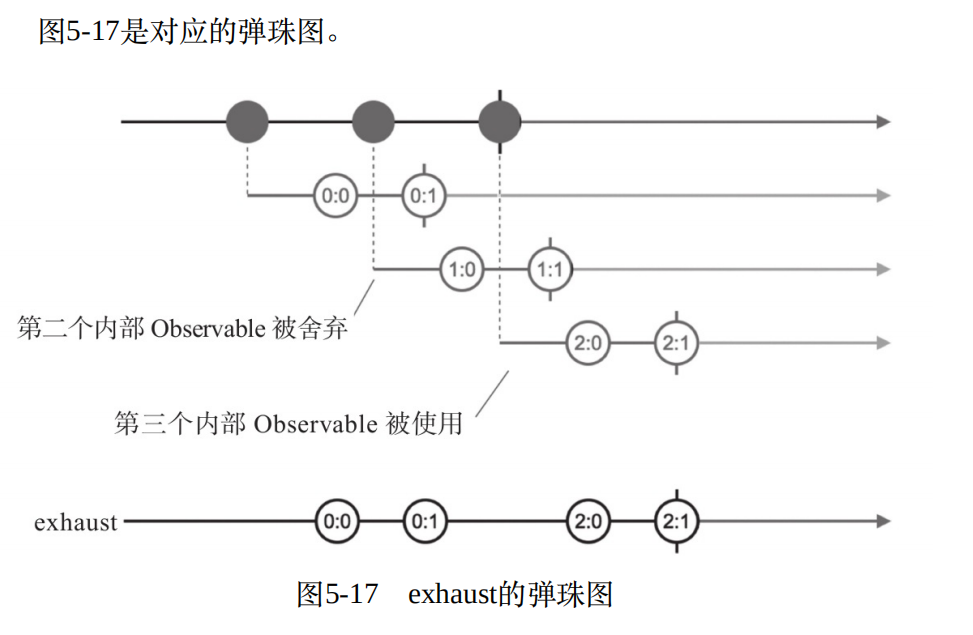posted on 2019-05-14 16:59 长安城下翩翩少年 阅读(...) 评论(...) 编辑 收藏